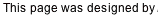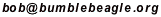## Hints

The row total for the fourth row is pretty high-- 73. The lowest number that could be in that line, and still allow a total of 73, would be 13. See if you can form any Christmassy four letter words from 13 thru 21 letters (O, G, Y, O, L, K, T, I, N), then check if these add up to 73. If you find one, you have the fourth row.

Another approach is geometric. Consider the numbers being added up when you add the totals 63, 75, 41, and 51. For example, 63 is the total of the label numbers on six ornaments. You may want to color those six ornaments lightly. Now do the same for the other three totals mentioned. You will have colored every ornament exactly once, except for the first ornament in the first row. This means you know the total of all the ornaments except that one. If you knew the total of all the ornaments, you could subtract this from it, and you'd know the number of the label that goes on that first ornament. There are a couple ways to get the total of all the ornaments. One is to add up all the numbers on the labels. Another is to add the four row totals.

Solution (don't look if you don't want to see the solution!)

Back to 1999 Christmas Puzzle